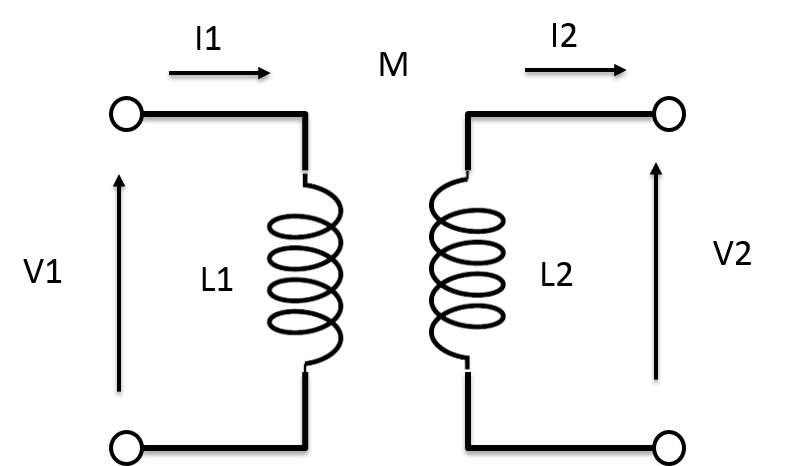Circuit theory is very good tool to understand and calculate the WPT system. In order to understand what happens in the magnetic coupling in circuit theory, two port network theory provides us good knowledge.

Let’s start discussion with the following figure. The figure shows the schematic of the 2-port network. the 2-port network will be anything such as band pass filter, transmission line or WPT system.We can describe this system with ABCD parameters. I recommend that you read the Pozar’s book to understand ABCD parameters first. One of the merits using ABCD parameters is that we can multiply each ABCD matrix when we deal with the cascade 2 port network systems.Before we go with the coupled inductors network, I would like to introduce three ABCD parameters of some typical 2 port network system.

(1) Series Impedance

(2) Shunt Impedance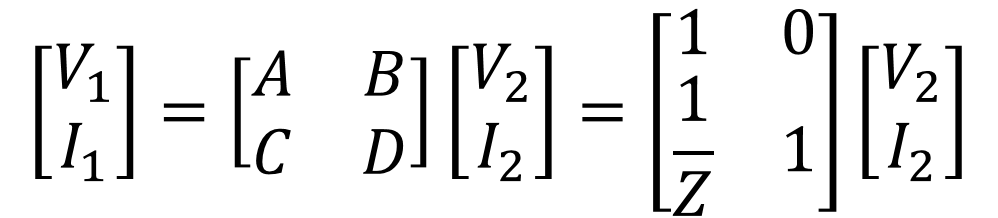(3) T-network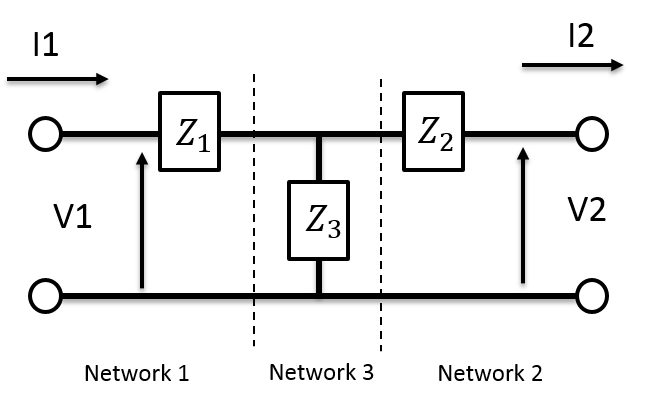We can see that T-network the cascade of three different networks. 1st and 2nd are the series impedance network while 3rd network is the shunt network.By using the rule of ABCD parameters, we can calculate the total ABCD parameters like the following equation.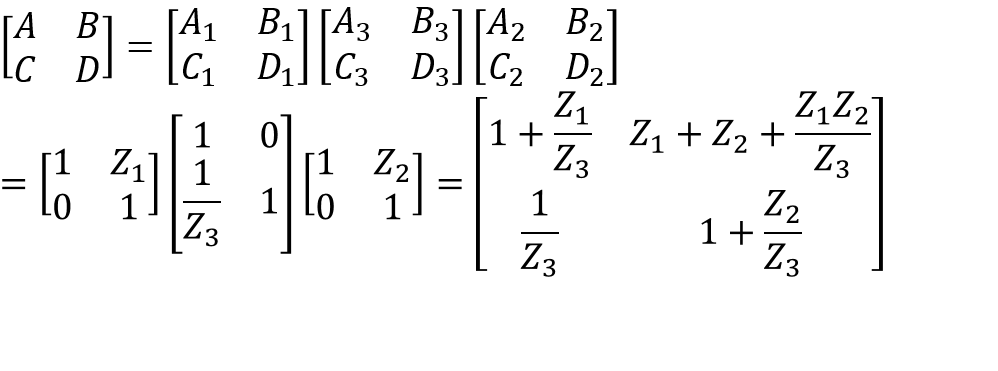Ok. Now we are ready to understand the coupled inductor network. Let us define the following system. The system consists of two coils and each inductance values are L1 and L2. The two coils are coupled with each other and the mutual inductance is shown to be M.Then we can convert this circuit diagram into equivalent circuit as the following figure. If you want to understand how we treat M in this equivalent circuit, you need to study the impedance converter. And you notice that the equivalent circuit is T networks of 2 port system. Please pay attention to how each impedance is defined at this system.Therefore, we can calculate the each ABCD parameters using the following equations.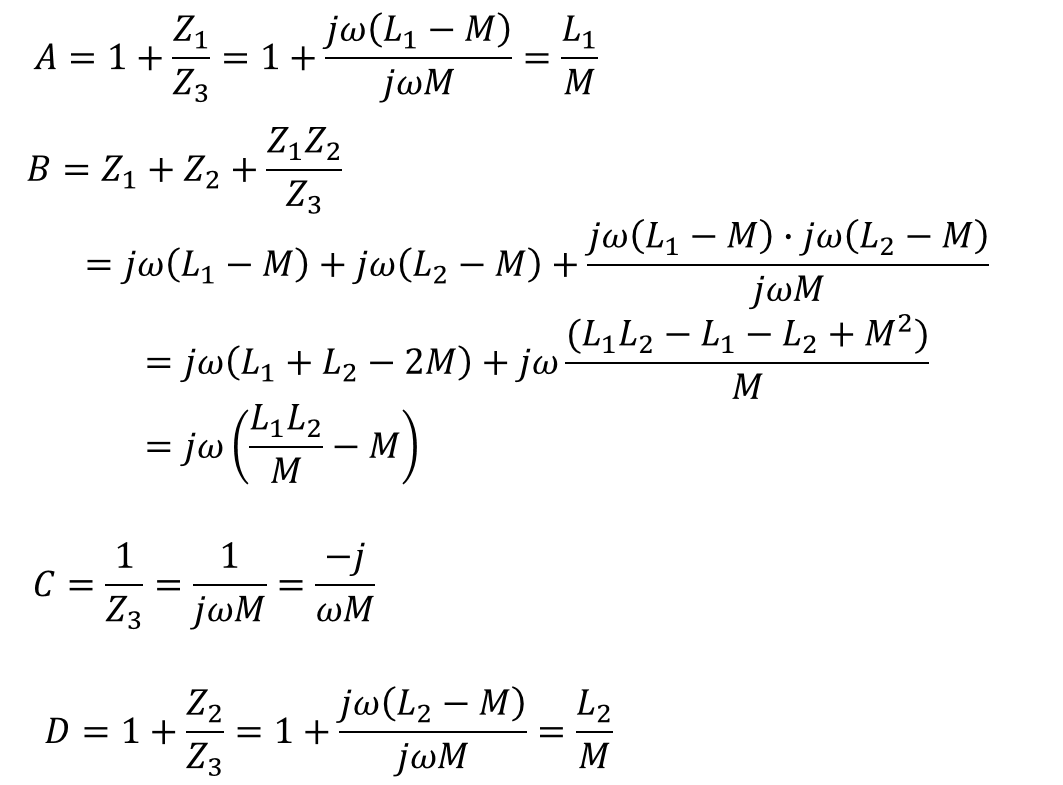The final ABCD matrix will be like the following equation.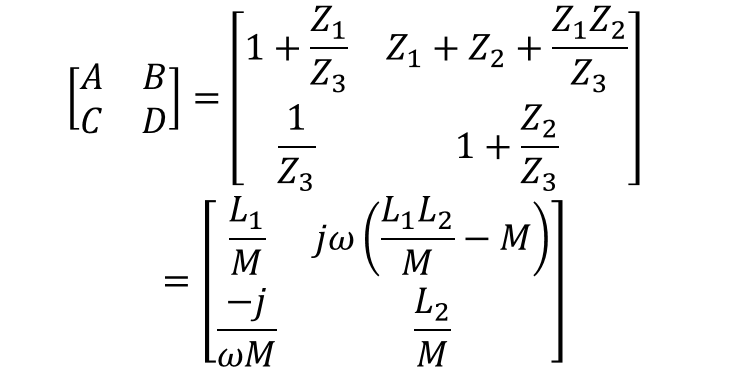To check the feasibility of this matrix, I would like to convert this ABCD parameters into Z parameters. and to compare the converted Z parameters with known values.

Let’s look at the following figures and how different Z parameter is with ABCD parameters.We have a very convenient table for converting each parameters.By using this conversion table, I would like to calculate the related Z parameters using ABCD parameters.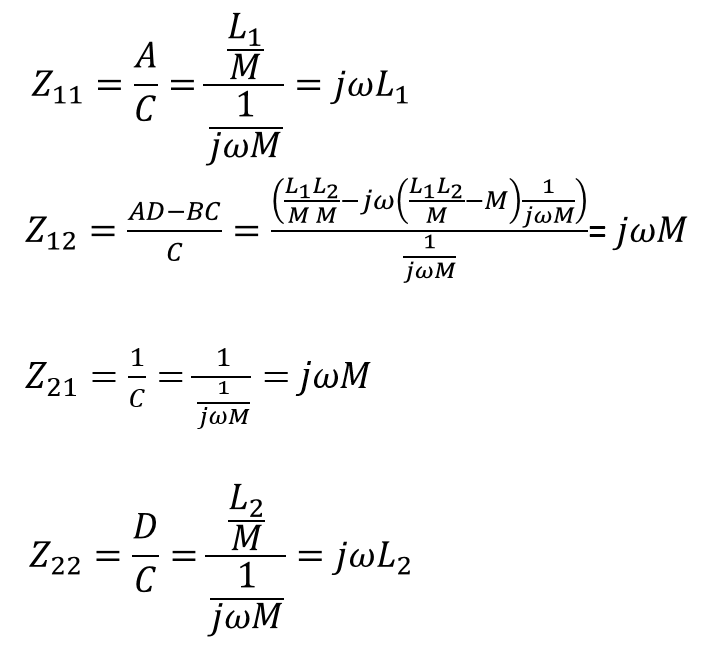The Z matrix will be as the following equation.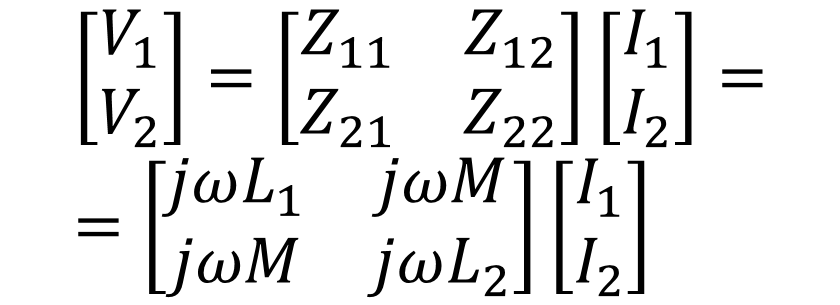This expression is the exactly with the same in Wikipedia’s database.https://en.wikipedia.org/wiki/Inductance

OK. If you understand this calculation, you are now in the half way to understand WPT system.  Thanks for your time.

## 1件のコメント

1. […]  http://wireless-square.com/2016/11/04/z-parameters-of-the-coupled-inductors-network/ […]

このサイトはスパムを低減するために Akismet を使っています。コメントデータの処理方法の詳細はこちらをご覧ください